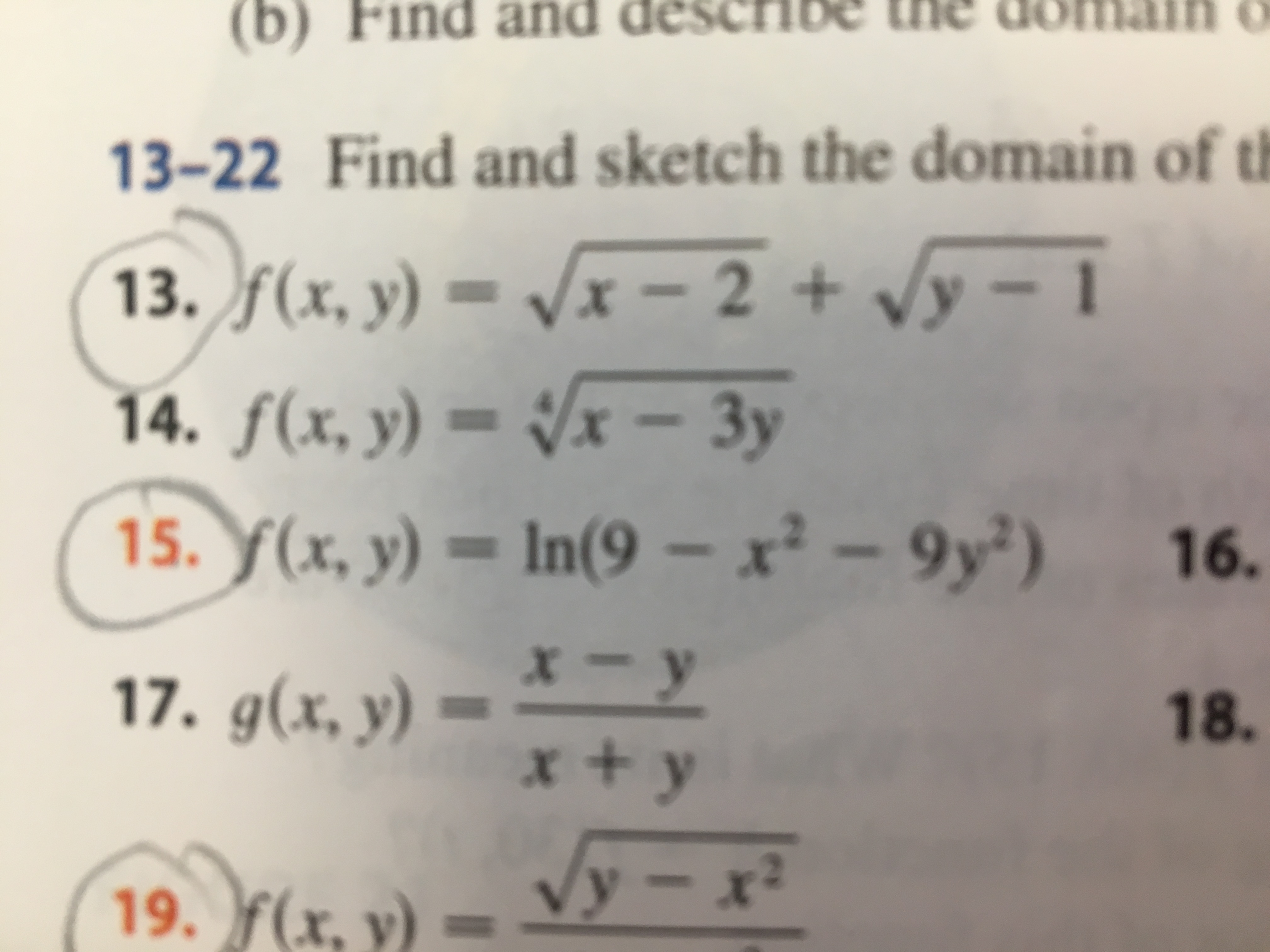# (b) Find and descrIBE the dO13-22 Find and sketch the domain of th.f(x, y) = Vx - 2 +y-114. f(x, y) = /x - 3yY(x, y) = In(9 - x - 9y2)XX16.x- yx + y17. g(x, y)18.19. f(x.y- x2

Question
3 views

#15help_outlineImage Transcriptionclose(b) Find and descrIBE the dO 13-22 Find and sketch the domain of th .f(x, y) = Vx - 2 +y-1 14. f(x, y) = /x - 3y Y(x, y) = In(9 - x - 9y2) X X 16. x- y x + y 17. g(x, y) 18. 19. f(x. y- x2 fullscreen
check_circle

star
star
star
star
star
1 Rating
Step 1

Given:

Step 2

To find the domain of the ...

### Want to see the full answer?

See Solution

#### Want to see this answer and more?

Solutions are written by subject experts who are available 24/7. Questions are typically answered within 1 hour.*

See Solution
*Response times may vary by subject and question.
Tagged in

### Functions